# Java基础第四天

## 1. 数组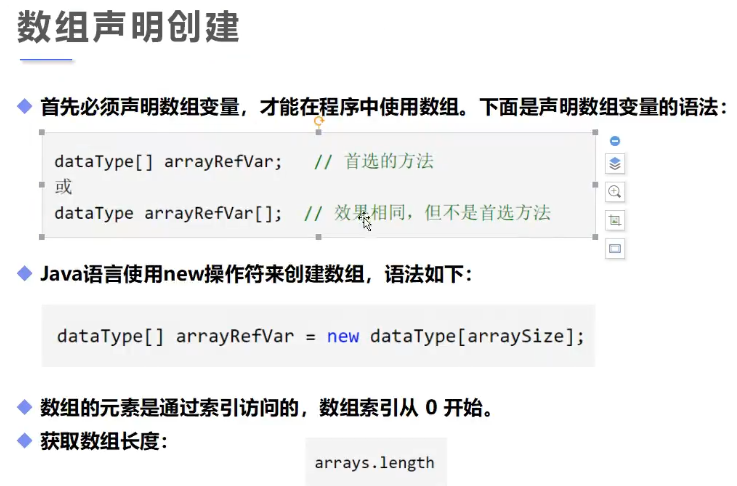### 1.1 数组的创建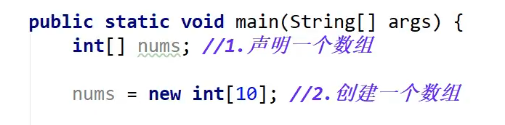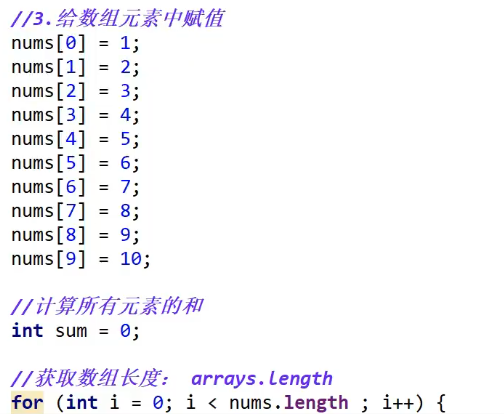### 1.2 数组内存关系（初步了解）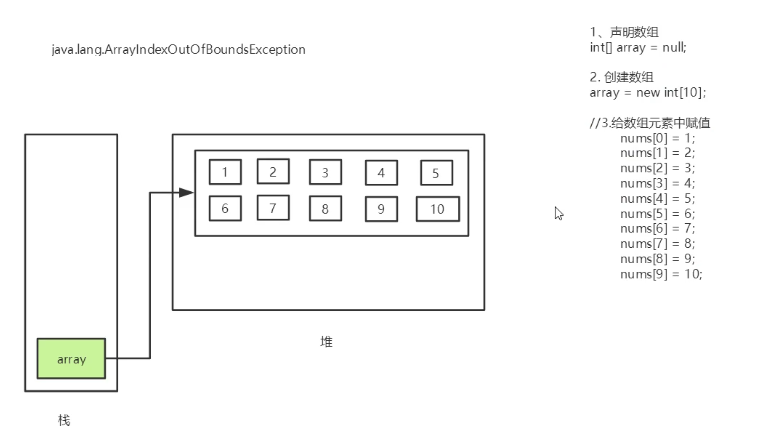（new关键字的东西都会放在堆里）

### 1.3 数组三种初始化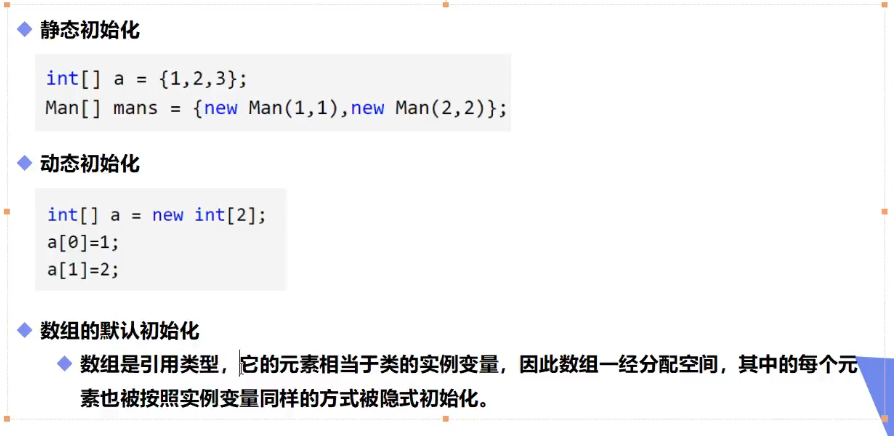### 1.4 数组的四个特点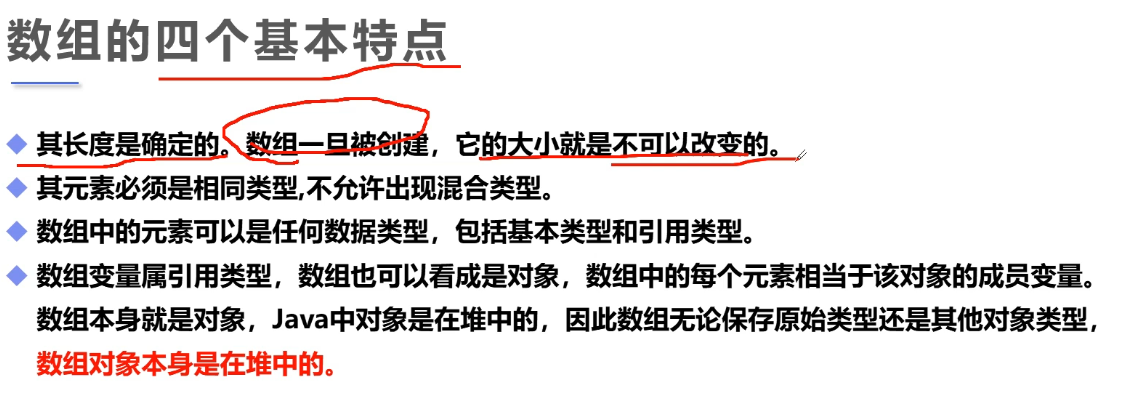### 1.5 数组边界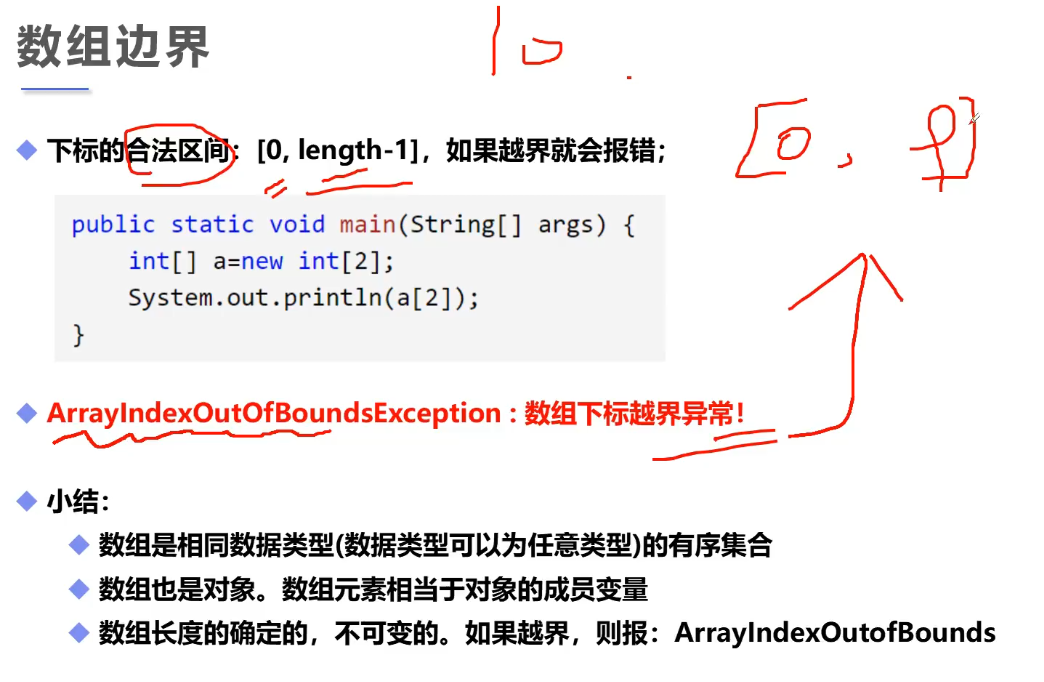### 1.6 数组的使用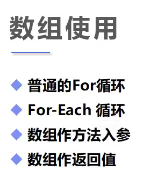public static void main(String[] args) {
int[] shuZu={1,2,3,4,5}; //静态初始化
int[] ints = fanZ(shuZu);
daYin2(ints);
}

public static int[] fanZ(int[] array) { //反转数组方法 （数组作为返回值和入参）
int[] result = new int[array.length]; //数组的长度为传入的长度
for (int i = 0; i<array.length; i++) { //遍历数组
result[i]=array[array.length-(i+1)];
}
return result;
}
public static void daYin(int[] array){
for (int i = 0; i < array.length; i++) {//普通for循环遍历
System.out.println(array[i]);
}
}

public static void daYin2(int[] array){
for (int i : array) { //for-each循环遍历
System.out.println(i); //i就相当于数组里的每个元素
}
}

### 1.7 多维数组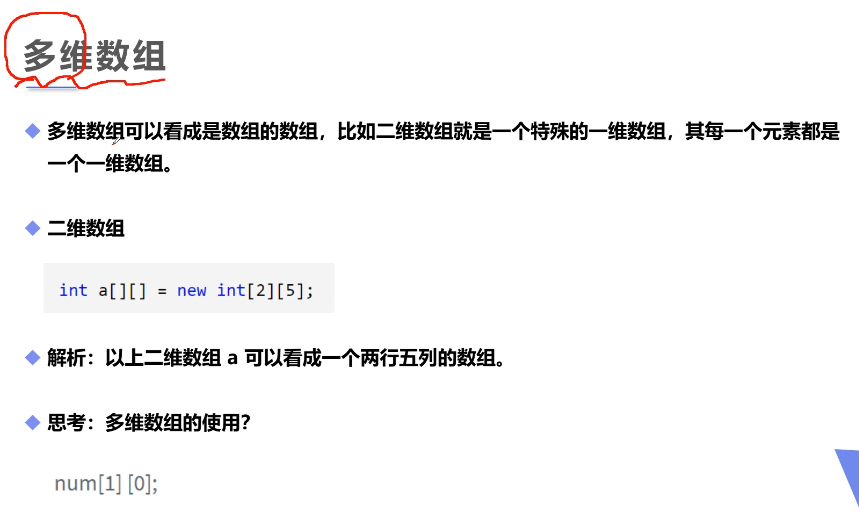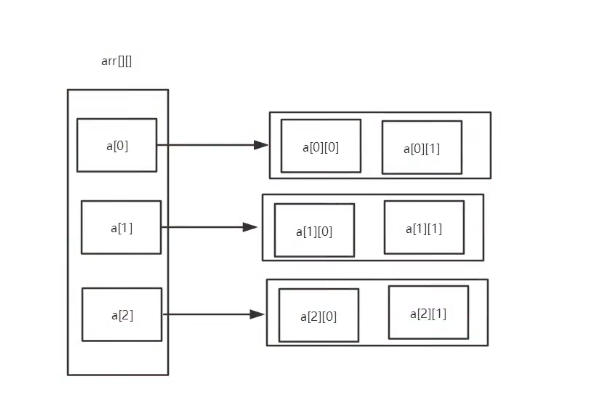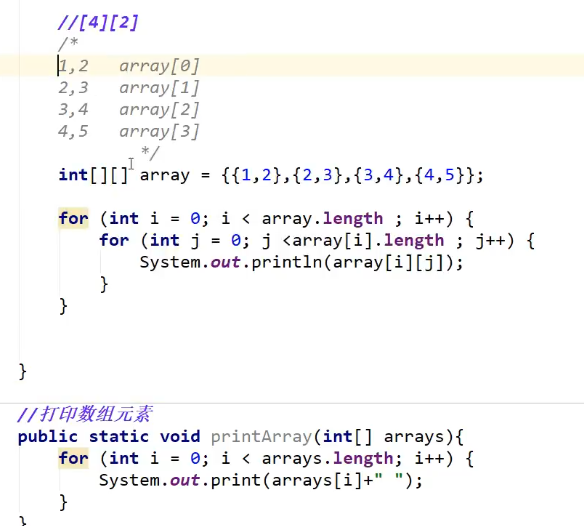### 1.8 Arrays类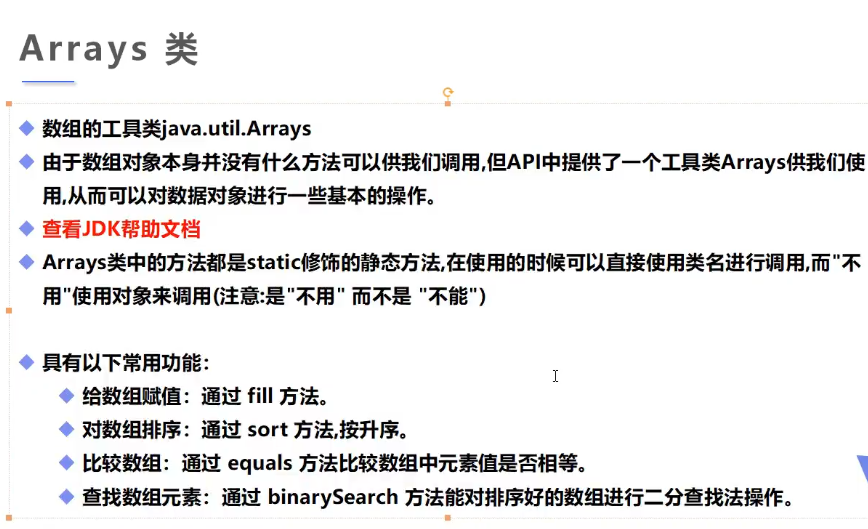### 1.9 冒泡排序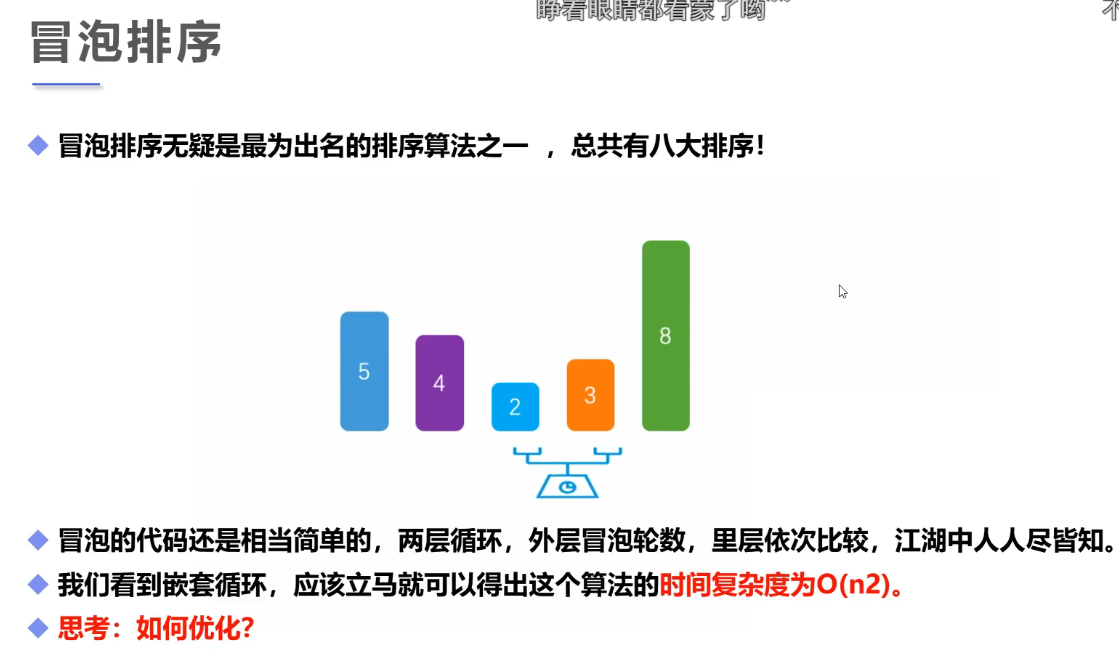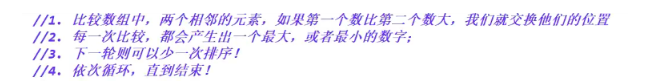int[] shu={5,10,2,8,90};
for (int i = 0; i < shu.length; i++) { //外层循环，判断一共要比较多少次
int temp;
boolean flag=false; //设定一个布尔值
for (int a = 0; a < shu.length-1; a++) { //内层循环
if (shu[a]>shu[a+1]){ //如果左边的值大于右边的
temp=shu[a+1]; //先把小的赋值给第三方
shu[a+1]=shu[a]; //把大的值赋值给小的
shu[a]=temp; //把第三方里小的值取出来给原先大的值
flag = true; //当有比较产生时就会进入该代码块，也就是还在进行比较，当没进来时说明比较已经完成，直接可以结束循环
}
}
if (flag == false){
break;
}

### 1.10 稀疏数组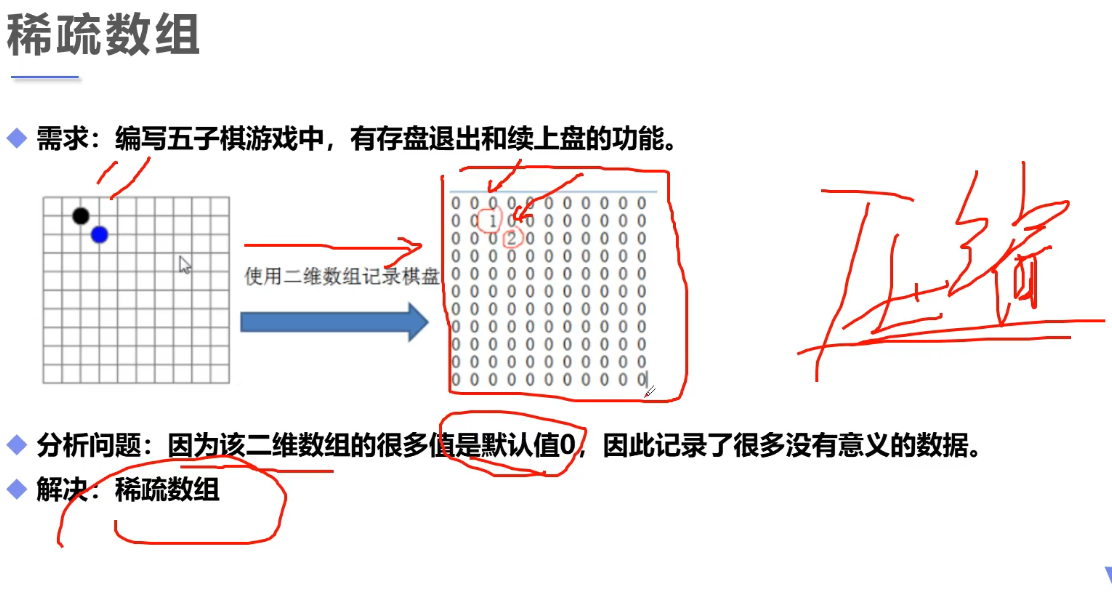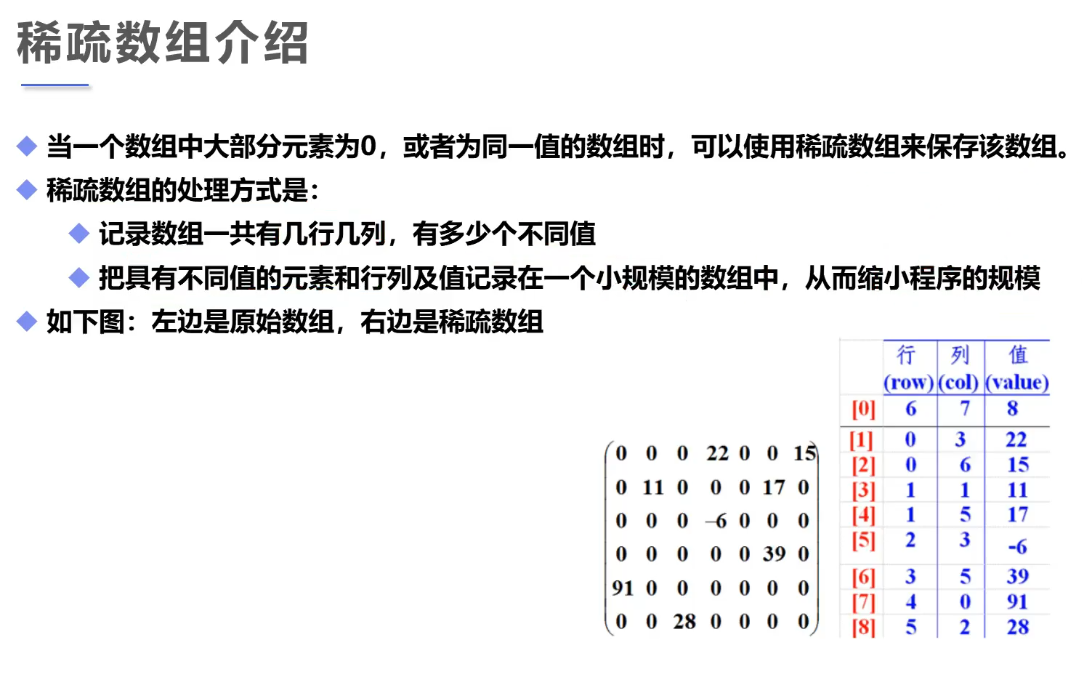public static void main(String[] args) {
int[][] qiPan=new int;
qiPan = 1;    qiPan = 2;
for (int i = 0; i < qiPan.length; i++) {
for (int q = 0; q < qiPan.length; q++) {
System.out.print(qiPan[i][q]+"  "); }
System.out.println(); }
//统计棋盘中的有效个数当作数组的行（列固定为3） ，有效个数+1 = 行数，因为第一行是固定存在
int sum=0;  //方便统计有效个数
for (int i = 0; i < qiPan.length; i++) {
for (int q = 0; q < qiPan[i].length; q++) {
if (qiPan[i][q] != 0){  //如果有值也就是不等于0 那么sum+1
sum++; } } }          //得到 有效个数后创建数组 （将棋盘转化为稀疏数组）
int[][] xiShu=new int[sum+1]; //（这里sum+1是因为除了存放有效值的行数之外还有一行存放固定值的列表头）
xiShu=11; //行固定是11
xiShu=11; //列固定是11
xiShu=sum; //有效个数为3
int count=0; //代表稀疏数组中的行数
for (int i = 0; i < qiPan.length; i++) {  //i可以用来代表行数
for (int q = 0; q < qiPan[i].length; q++) { //q可以用来代表列数_qiPan[i].length代表这里边每个数组中的数组的长度
if (qiPan[i][q]!=0){ //如果有值也就是不等于0
count++; //如果有值那么++ 代表行数+1
xiShu[count] = i; //稀疏数列的 第count行的行的值就是i
xiShu[count] = q; //稀疏数列的 第count行的列的值就是q
xiShu[count] = qiPan[i][q]; }}}//疏数列的 第count行的值的值就是qiPan[i][q]
System.out.println("========================"); System.out.println("输出稀疏数组:");
for (int[] ints : xiShu) {
for (int anInt : ints) {
System.out.print(anInt+" "); }  System.out.println(" "); }
System.out.println("还原稀疏数组:");
int[][] huanYuan= new int[xiShu][xiShu];
for (int i = 1; i < xiShu.length; i++) { //从第二行开始遍历，因为第一行是头信息
huanYuan[xiShu[i]][xiShu[i]] = xiShu[i];  //xiShu[i] 代表的是行数，
// [xiShu[i]代表的是列数 将xiShu[i] 赋值给huanYuan数组的这一行这一列
}
for (int[] ints : huanYuan) {
for (int anInt : ints) {
System.out.print(anInt+"  ");
}
System.out.println(" ");
}
}